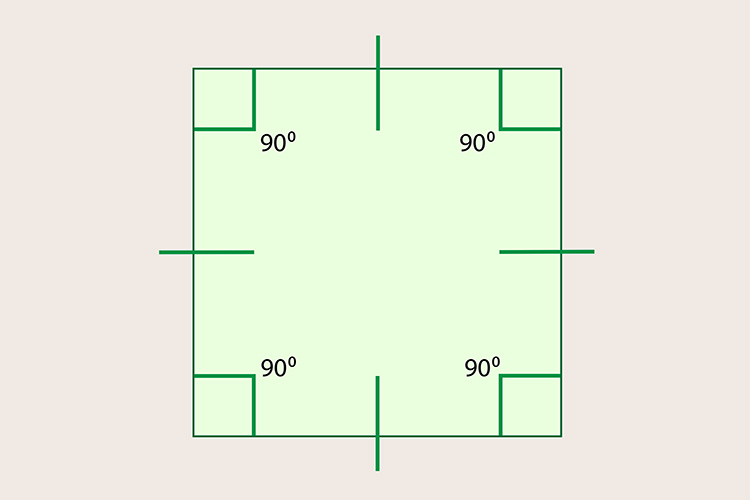# The total interior angles of a square (or rectangle) = 360°

Each time we add a side (triangle to square, square to pentagon, pentagon to hexagon), we add another 180°. Triangle to square =180°+180°=360°1 2 3 4 90° + 90° + 90° + 90° = 360°

The total internal angles of a square = 360°